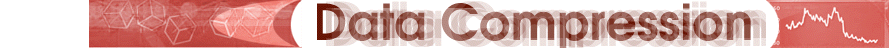Introduction Algorithm     - DCT     - Coefficient Quantization     - Lossless Compression Color Future Lossless Compression of Quantized ValuesThe final step of the JPEG image compression process is to compress the quantized DCT values. This is done through a three-part procedure detailed below. 1. Convert the DC coefficient to a relative value – First, the DC coefficient is changed from an absolute value to a relative value – relative to the DC coefficient in the previous 8 x 8 block, that is. Since adjacent blocks in an image exhibit a high degree of correlation, coding the DC term of a given block as the difference from the previous DC term typically produces a very small number that can be stored in a fewer number of bits. 2. Reorder the DCT block in a zig-zag sequence – Because so many coefficients in the DCT image are truncated to zero values during the coefficient quantization stage, the zeros are handled differently than non-zero coefficients. They are coded using a Run-Length Encoding (RLE) algorithm. RLE gives a count of consecutive zero values in the image, and the longer the runs of zeros, the greater the compression. One way to increase the length of runs is to reorder the coefficients in the zig-zag sequence shown in the diagram below. This way, the JPEG algorithm moves through the block selecting the highest value elements first and eventually working its way to the lowest value elements, thus optimizing the effect of RLE. 3. Entropy Encoding – Finally, the JPEG algorithm outputs the DCT block’s elements using an entropy encoding mechanism that combines the principles of RLE and Huffman encoding. The output of the entropy encoder consists of a sequence of three tokens, repeated until the block is complete. The three tokens are the run length, the number of consecutive zeros that precede the current non-zero element in the DCT output matrix; the bit count, the number of bits used to encode the amplitude value that follows, as determined by the Huffman encoding scheme; and the amplitude, the amplitude of the DCT coefficient.The final output that results from JPEG compression can be mathematically reversed and decompressed to produce an image that, to a computer, lacks defining data, but to a human eye, appears identical to the original image.